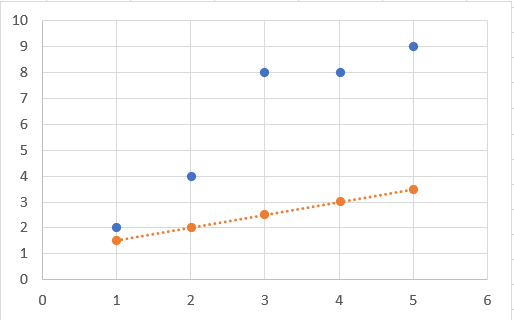1596809319

# Linear Regression From Scratch Using Gradient Descent

In this video, I am gonna implement linear regression from scratch without any ML libraries

https://youtu.be/vmS3Z70hyyQ

## Buddha Community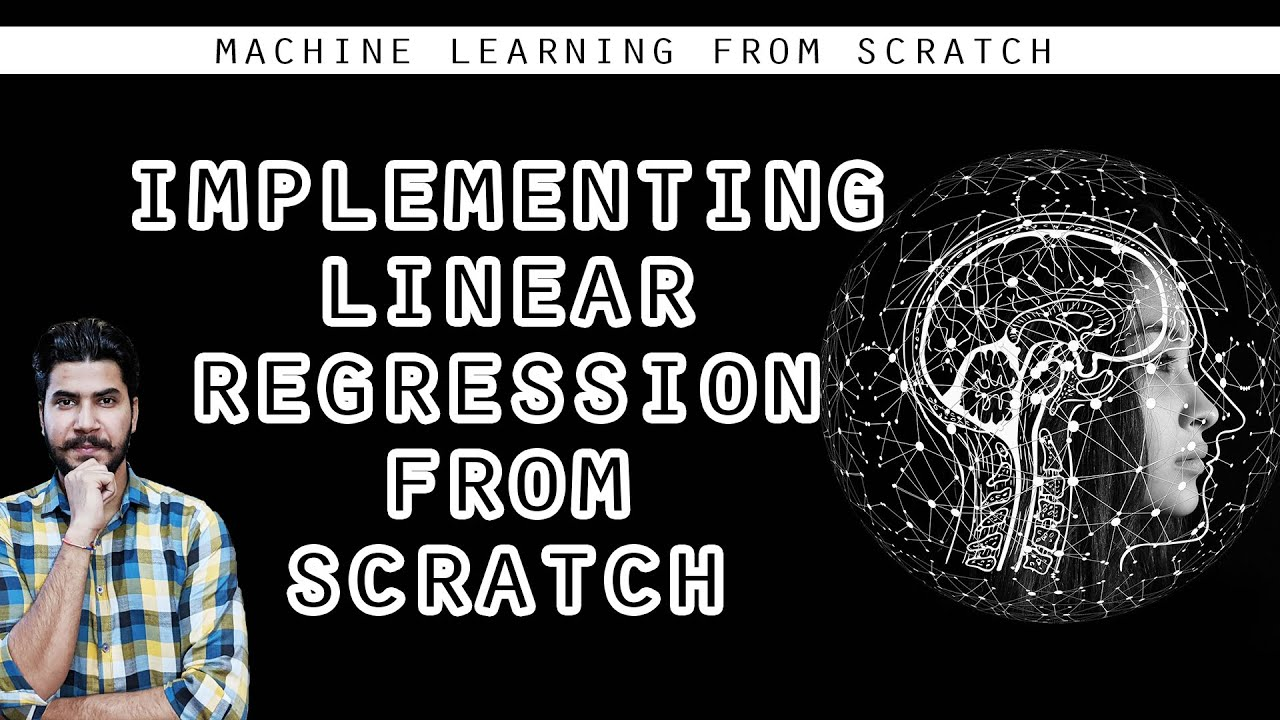1603753200

## ML Optimization pt.1 - Gradient Descent with Python

So far in our journey through the Machine Learning universe, we covered several big topics. We investigated some regression algorithms, classification algorithms and algorithms that can be used for both types of problems (SVM**, **Decision Trees and Random Forest). Apart from that, we dipped our toes in unsupervised learning, saw how we can use this type of learning for clustering and learned about several clustering techniques.

We also talked about how to quantify machine learning model performance and how to improve it with regularization. In all these articles, we used Python for “from the scratch” implementations and libraries like TensorFlowPytorch and SciKit Learn. The word optimization popped out more than once in these articles, so in this and next article, we focus on optimization techniques which are an important part of the machine learning process.

In general, every machine learning algorithm is composed of three integral parts:

1. loss function.
2. Optimization criteria based on the loss function, like a cost function.
3. Optimization technique – this process leverages training data to find a solution for optimization criteria (cost function).

As you were able to see in previous articles, some algorithms were created intuitively and didn’t have optimization criteria in mind. In fact, mathematical explanations of why and how these algorithms work were done later. Some of these algorithms are Decision Trees and kNN. Other algorithms, which were developed later had this thing in mind beforehand. SVMis one example.

During the training, we change the parameters of our machine learning model to try and minimize the loss function. However, the question of how do you change those parameters arises. Also, by how much should we change them during training and when. To answer all these questions we use optimizers. They put all different parts of the machine learning algorithm together. So far we mentioned Gradient Decent as an optimization technique, but we haven’t explored it in more detail. In this article, we focus on that and we cover the grandfather of all optimization techniques and its variation. Note that these techniques are not machine learning algorithms. They are solvers of minimization problems in which the function to minimize has a gradient in most points of its domain.

### Dataset & Prerequisites

Data that we use in this article is the famous Boston Housing Dataset . This dataset is composed 14 features and contains information collected by the U.S Census Service concerning housing in the area of Boston Mass. It is a small dataset  with only 506 samples.For the purpose of this article, make sure that you have installed the following _Python _libraries:

• **NumPy **– Follow this guide if you need help with installation.
• **SciKit Learn **– Follow this guide if you need help with installation.
• Pandas – Follow this guide if you need help with installation.

Once installed make sure that you have imported all the necessary modules that are used in this tutorial.

``````import pandas as pd
import numpy as np
import matplotlib.pyplot as plt

from sklearn.model_selection import train_test_split
from sklearn.metrics import mean_squared_error
from sklearn.preprocessing import StandardScaler
from sklearn.linear_model import SGDRegressor
``````

Apart from that, it would be good to be at least familiar with the basics of linear algebracalculus and probability.

### Why do we use Optimizers?

Note that we also use simple Linear Regression in all examples. Due to the fact that we explore optimizationtechniques, we picked the easiest machine learning algorithm. You can see more details about Linear regression here. As a quick reminder the formula for linear regression goes like this: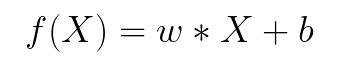where w and b are parameters of the machine learning algorithm. The entire point of the training process is to set the correct values to the w and b, so we get the desired output from the machine learning model. This means that we are trying to make the value of our error vector as small as possible, i.e. to find a global minimum of the cost function.

One way of solving this problem is to use calculus. We could compute derivatives and then use them to find places where is an extrema of the cost function. However, the cost function is not a function of one or a few variables; it is a function of all parameters of a machine learning algorithm, so these calculations will quickly grow into a monster. That is why we use these optimizers.

#ai #machine learning #python #artificaial inteligance #artificial intelligence #batch gradient descent #data science #datascience #deep learning #from scratch #gradient descent #machine learning #machine learning optimizers #ml optimization #optimizers #scikit learn #software #software craft #software craftsmanship #software development #stochastic gradient descent1603033200

## A Complete Guide to Linear Regression for Beginners

What is supervised learning? In supervised learning, you have an input-output pair. And you will try to map the given input to output by training the input-output pair.

Another type of machine learning algorithm is unsupervised learning, in this, you don’t have an output variable. You will try to group the input variables by their similarities.

What is Regression? Regression is a statistical process of estimating the relationship between a dependant variable and several independent variables.

In other words, it can be said that linear regression suggests that the output variable can be represented as a linear combination of the input variables.

Linear Regression Example

Depending upon the number of input variables, linear regression can be classified into simple and multiple linear regression. If the number of input variables is one, then it is called simple linear regression.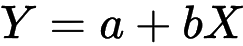Simple Linear Regression Formula

If there is more than one input variable, then it is called multiple linear regression.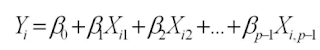Multiple Linear Regression Formula

In this blog post, I will be discussing simple linear regression.1594271340

## A Deep Dive into Linear Regression

Let’s begin our journey with the truth — machines never learn. What a typical machine learning algorithm does is find a mathematical equation that, when applied to a given set of training data, produces a prediction that is very close to the actual output.

Why is this not learning? Because if you change the training data or environment even slightly, the algorithm will go haywire! Not how learning works in humans. If you learned to play a video game by looking straight at the screen, you would still be a good player if the screen is slightly tilted by someone, which would not be the case in ML algorithms.

However, most of the algorithms are so complex and intimidating that it gives our mere human intelligence the feel of actual learning, effectively hiding the underlying math within. There goes a dictum that if you can implement the algorithm, you know the algorithm. This saying is lost in the dense jungle of libraries and inbuilt modules which programming languages provide, reducing us to regular programmers calling an API and strengthening further this notion of a black box. Our quest will be to unravel the mysteries of this so-called ‘black box’ which magically produces accurate predictions, detects objects, diagnoses diseases and claims to surpass human intelligence one day.

We will start with one of the not-so-complex and easy to visualize algorithm in the ML paradigm — Linear Regression. The article is divided into the following sections:

1. Need for Linear Regression

2. Visualizing Linear Regression

3. Deriving the formula for weight matrix W

4. Using the formula and performing linear regression on a real world data set

Note: Knowledge on Linear Algebra, a little bit of Calculus and Matrices are a prerequisite to understanding this article

Also, a basic understanding of python, NumPy, and Matplotlib are a must.

## 1) Need for Linear regression

Regression means predicting a real valued number from a given set of input variables. Eg. Predicting temperature based on month of the year, humidity, altitude above sea level, etc. Linear Regression would therefore mean predicting a real valued number that follows a linear trend. Linear regression is the first line of attack to discover correlations in our data.

Now, the first thing that comes to our mind when we hear the word linear is, a line.

Yes! In linear regression, we try to fit a line that best generalizes all the data points in the data set. By generalizing, we mean we try to fit a line that passes very close to all the data points.

But how do we ensure that this happens? To understand this, let’s visualize a 1-D Linear Regression. This is also called as Simple Linear Regression

#calculus #machine-learning #linear-regression-math #linear-regression #linear-regression-python #python1594890120

## Linear Regression — Part II — Gradient Descent

Gradient descent_ is an optimization algorithm used to minimize a cost function (i.e. Error) parameterized by a model._

We know that Gradient means the slope of a surface or a line. This algorithm involves calculations with slope.

To understand about Gradient Descent, we must know what is a cost function.

Cost function(J) of Linear Regression is the Root Mean Squared Error (RMSE) between predicted y value (predicted) and true y value (y).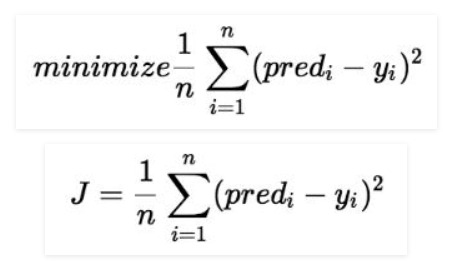For a Linear Regression model, our ultimate goal is to get the minimum value of a Cost Function.

First Let us visualize how the Gradient Descent looks like to understand better.

As Gradient Descent is an iterative algorithm, we will be fitting various lines to find the best fit line iteratively.

Each time we get an error value (SSE).

If we fit all the error values in a graph, it will become a parabola.

W

hat is the Relationship between Slope, Intercept and SSE? Why Gradient Descent is a Parabola?

To answer these 2 questions, Let’s see the below example.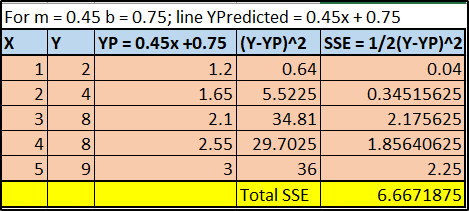You can notice that I took random value of m = 0.45 & c = 0.75.

For this slope and intercept we have come up with a new Y predicted values for a regression line.